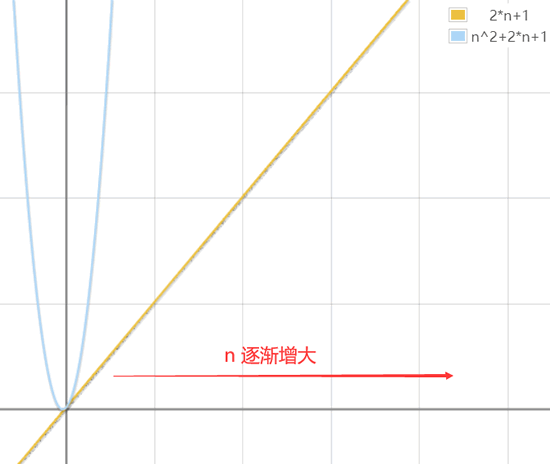##MSIPO技术圈 首页 IT技术 查看内容

# 数据结构与算法——时间复杂度和空间复杂度（详解版）

2023-07-13## “好”算法的标准

• 程序的运行时间。
• 程序运行所需内存空间的大小。

## 时间复杂度

``````for(int i = 0 ; i < n ; i++)     //<- 从 0 到 n，执行 n+1 次
{
a++;                         //<- 从 0 到 n-1，执行 n 次
}``````

• for 循环从 i 的值为 0 一直逐增至 n（注意，循环退出的时候 i 值为 n），因此 for 循环语句执行了 n+1 次；
• 而循环内部仅有一条语句，a++ 从 i 的值为 0 就开始执行，i 的值每增 1 该语句就执行一次，一直到 i 的值为 n-1，因此，a++ 语句一共执行了 n 次。

``````for(int i = 0 ; i < n ; i++)           // n+1
{
for(int j = 0 ; j < m ; j++)       // n*(m+1)
{
num++;                         // n*m
}
}``````• 首先，常数 1 是可以忽略不计的；
• 其次，对于指数级的 2*n2 来说，是否在其基础上加 2*n，并无关紧要；
• 甚至于，对于是否给 n2 乘 2，也可以忽略。

• 去掉频度表达式中，所有的加法常数式子。例如 2n2+2n+1 简化为 2n2+2n ；
• 如果表达式有多项含有无限大变量的式子，只保留一个拥有指数最高的变量的式子。例如 2n2+2n 简化为 2n2；
• 如果最高项存在系数，且不为 1，直接去掉系数。例如 2n2 系数为 2，直接简化为 n2 ；

``O(频度)``

``O(1)常数阶 < O(logn)对数阶 < O(n)线性阶 < O(n2)平方阶 < O(n3)(立方阶) < O(2n) (指数阶)``

## 空间复杂度

• 程序代码本身所占用的存储空间；
• 程序中如果需要输入输出数据，也会占用一定的存储空间；
• 程序在运行过程中，可能还需要临时申请更多的存储空间。

``````int n;
scanf("%d", &n);
int a;``````

``int a[n];``

• 如果随着输入值 n 的增大，程序申请的临时空间成线性增长，则程序的空间复杂度用 O(n) 表示;
• 如果随着输入值 n 的增大，程序申请的临时空间成 n2 关系增长，则程序的空间复杂度用 O(n2) 表示；
• 如果随着输入值 n 的增大，程序申请的临时空间成 n3 关系增长，则程序的空间复杂度用 O(n3) 表示；
• 等等。